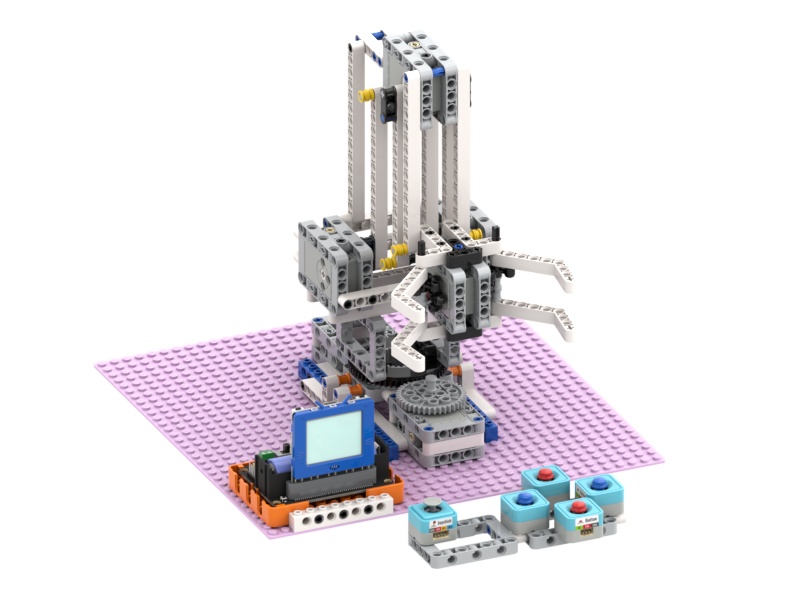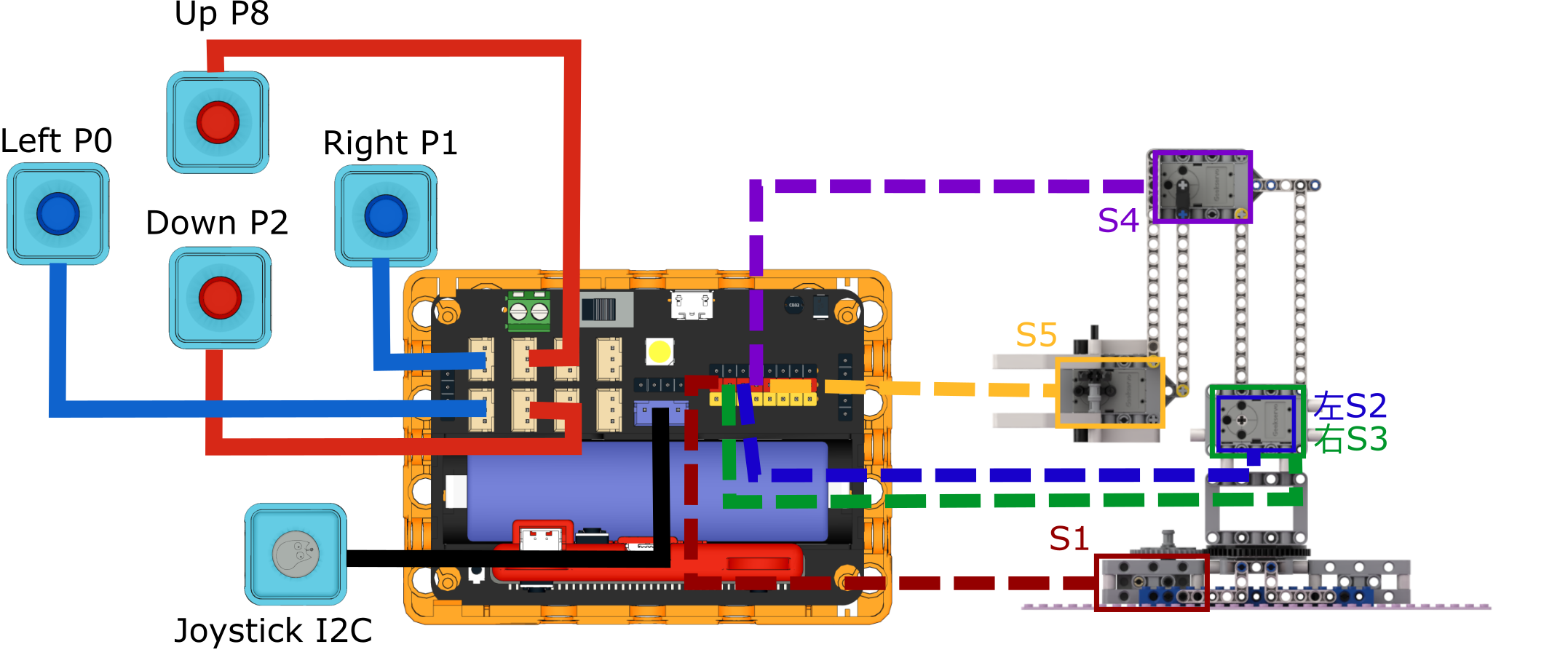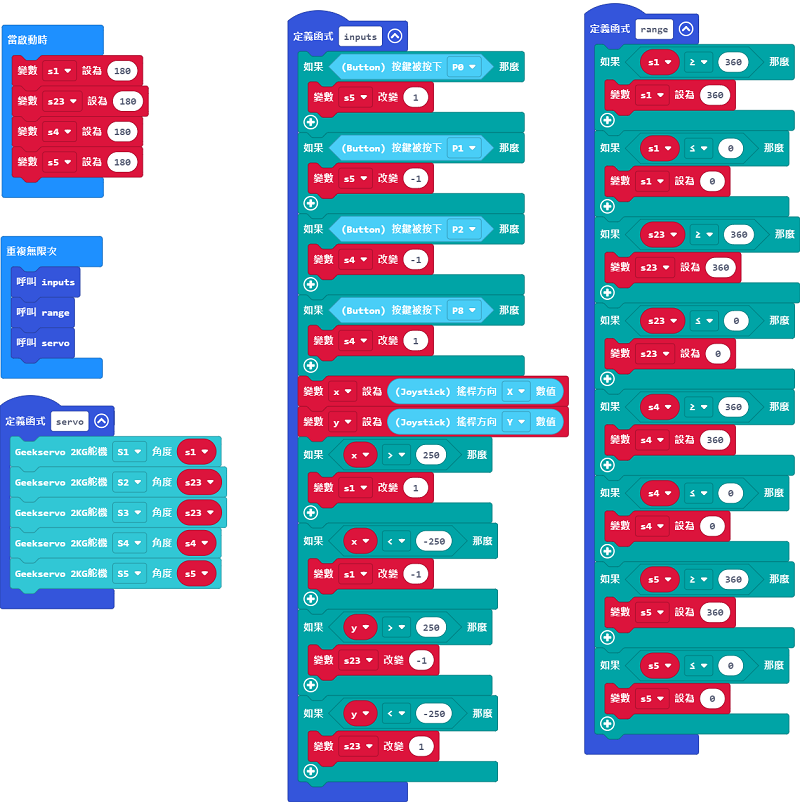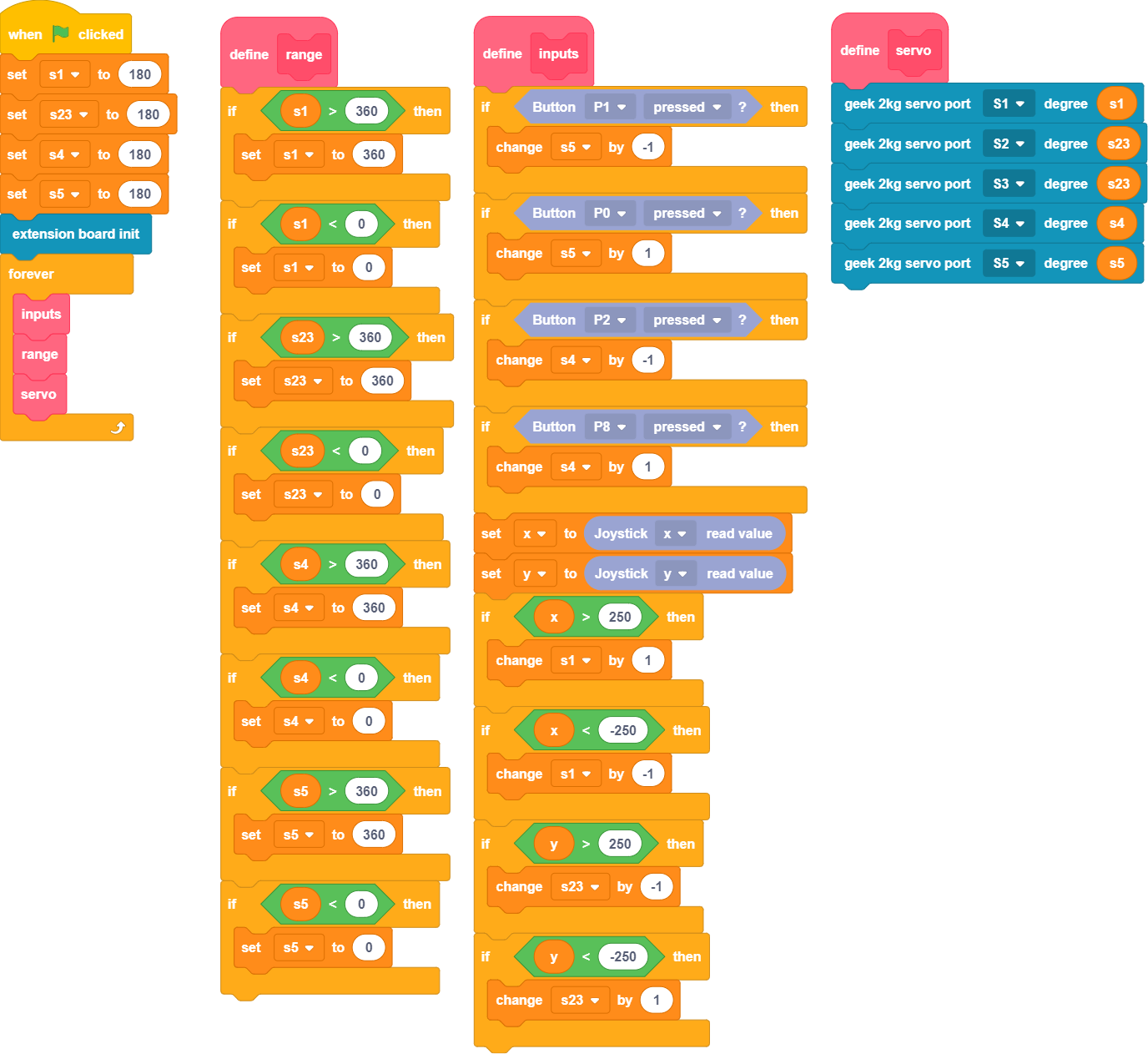# Industry 4.0 Robotic ArmThis Robotic Arm is built of plastic building blocks, it features 5 Geekservo 2KG servos.

## Kit Contents

1. Robotbit Edu x1

2. 18650 Lithium Battery x1

3. Geekservo 2KG Servos x5

4. Sugar Button Modules x4

5. Sugar Joystick Module x1

6. Building Blocks Set

7. Connecting Cables

## Features

• Mechanical structure allows students to learn mechanical engineering concepts

• Improving engineering skills by hands-on construction of the robot arm

• Brick-built structure allows flexibility in modifying to suit different scenarios

## Sample Wiring Diagram

### Robotbit EDU## Sample Program

### Micro:bit Sample ProgramSample Program

### KittenBlock Sample ProgramSample Program(Right Click->Save As)

### Python Sample Program

```#/bin/python

from future import *
from sugar import *
import robotbit

x = 0
s1 = 0
s23 = 0
s4 = 0
s5 = 0
y = 0

def inputs():
global x,s1,s23,s4,s5,y

if Button("P1").value() == 0:
s5 += -1
if Button("P0").value() == 0:
s5 += 1
if Button("P2").value() == 0:
s4 += -1
if Button("P8").value() == 0:
s4 += 1
x = Joystick().value('x')
y = Joystick().value('y')
if x > 250:
s1 += 1
if x < -250:
s1 += -1
if y > 250:
s23 += -1
if y < -250:
s23 += 1

def range():
global x,s1,s23,s4,s5,y

if s1 > 360:
s1 = 360
if s1 < 0:
s1 = 0
if s23 > 360:
s23 = 360
if s23 < 0:
s23 = 0
if s4 > 360:
s4 = 360
if s4 < 0:
s4 = 0
if s5 > 360:
s5 = 360
if s5 < 0:
s5 = 0

def servo():
global x,s1,s23,s4,s5,y
robot.geekServo2kg(1, s1)
robot.geekServo2kg(2, s23)
robot.geekServo2kg(3, s23)
robot.geekServo2kg(4, s4)
robot.geekServo2kg(5, s5)

s1 = 180
s23 = 180
s4 = 180
s5 = 180

robot = robotbit.RobotBit()
while True:
inputs()
range()
servo()
```

Sample Program(Right Click->Save As)